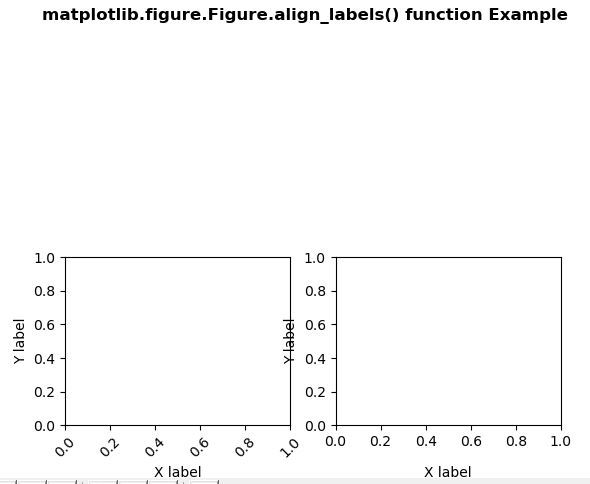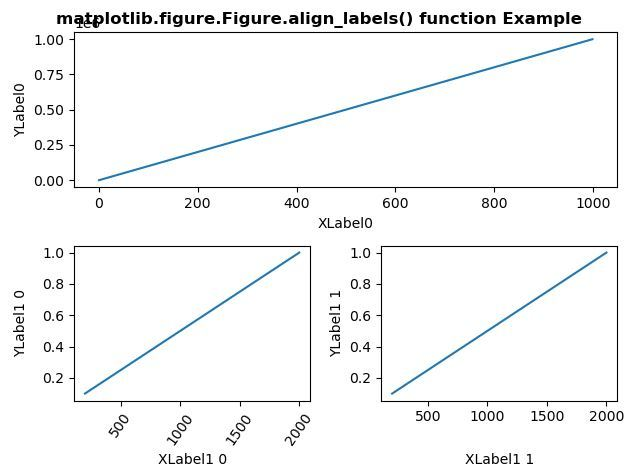# Matplotlib.figure.Figure.align_labels() in Python

Matplotlib is a library in Python and it is numerical – mathematical extension for NumPy library. The figure module provides the top-level Artist, the Figure, which contains all the plot elements. This module is used to control the default spacing of the subplots and top level container for all plot elements.

## matplotlib.figure.Figure.align_labels() function

The align_labels() method figure module of matplotlib library is used to Align the xlabels and ylabels of subplots with the same subplots row or column if label alignment is being done automatically.

Syntax: align_labels(self, axs=None)

Parameters: This accept the following parameters that are described below:

• axs : This parameter is the list of Axes to align the labels.

Returns: This method does not return any value.

Below examples illustrate the matplotlib.figure.Figure.align_labels() function in matplotlib.figure:

Example 1:

 `# Implementation of matplotlib function ` `import` `matplotlib.pyplot as plt ` `import` `numpy as np ` `import` `matplotlib.gridspec as gridspec ` ` `  `fig ``=` `plt.figure() ` `gs ``=` `gridspec.GridSpec(``2``, ``2``) ` ` `  `for` `i ``in` `range``(``2``): ` `    ``ax ``=` `fig.add_subplot(gs[``1``, i]) ` `    ``ax.set_ylabel(``'Y label'``) ` `    ``ax.set_xlabel(``'X label'``) ` `    ``if` `i ``=``=` `0``: ` `        ``for` `tick ``in` `ax.get_xticklabels(): ` `            ``tick.set_rotation(``45``) ` ` `  `fig.align_labels() ` ` `  `fig.suptitle('matplotlib.figure.Figure.align_labels() \ ` `function Example\n\n', fontweight ``=``"bold"``) ` ` `  `plt.show() `

Output:Example 2:

 `# Implementation of matplotlib function ` `import` `matplotlib.pyplot as plt ` `import` `numpy as np ` `import` `matplotlib.gridspec as gridspec ` ` `  `fig ``=` `plt.figure(tight_layout ``=` `True``) ` `gs ``=` `gridspec.GridSpec(``2``, ``2``) ` ` `  `ax ``=` `fig.add_subplot(gs[``0``, :]) ` `ax.plot(np.arange(``0``, ``1e6``, ``1000``)) ` ` `  `ax.set_ylabel(``'YLabel0'``) ` `ax.set_xlabel(``'XLabel0'``) ` ` `  `for` `i ``in` `range``(``2``): ` `    ``ax ``=` `fig.add_subplot(gs[``1``, i]) ` `    ``ax.plot(np.arange(``1.``, ``0.``, ``-``0.1``) ``*` `2000.``, ` `             `  `            ``np.arange(``1.``, ``0.``, ``-``0.1``)) ` `     `  `    ``ax.set_ylabel(``'YLabel1 % d'` `%` `i) ` `    ``ax.set_xlabel(``'XLabel1 % d'` `%` `i) ` `     `  `    ``if` `i ``=``=` `0``: ` `        ``for` `tick ``in` `ax.get_xticklabels(): ` `            ``tick.set_rotation(``55``) ` ` `  `fig.align_labels()   ` ` `  `fig.suptitle('matplotlib.figure.Figure.align_labels() \ ` `function Example\n\n', fontweight ``=``"bold"``) ` ` `  `plt.show() `

Output:My Personal Notes arrow_drop_upCheck out this Author's contributed articles.

If you like GeeksforGeeks and would like to contribute, you can also write an article using contribute.geeksforgeeks.org or mail your article to contribute@geeksforgeeks.org. See your article appearing on the GeeksforGeeks main page and help other Geeks.

Please Improve this article if you find anything incorrect by clicking on the "Improve Article" button below.

Article Tags :

Be the First to upvote.

Please write to us at contribute@geeksforgeeks.org to report any issue with the above content.